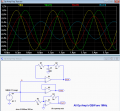# Open loop gain and frequency response

#### Nemanja_95

Joined Nov 14, 2017
24

#### crutschow

Joined Mar 14, 2008
33,378
Depends upon the gain-bandwidth of the op amp.
Open-loop the gain will be about 1 at the gain-bandwidth product frequency.

But note that you can't reliably test the open loop response as you show because of offsets which will drive a real op amp to one of the output rails.
You need to provide low frequency DC feedback to stabilize the operating point.

Below is the simulation for an ideal op amp with a 1MHz gain-bandwidth and a 1MHz input .
Out1 is the open loop response, Out2 is the response for a gain of +1 , and Out3 is response for a gain of -1 .

I'll leave it to you to explain the differences.Last edited:

#### Nemanja_95

Joined Nov 14, 2017
24
So, out1 is the same amplitude as input signal because it is open loop op amp at its gain-bandwidth product frequency (gain about 1).
Out2 is little lower amplitude because at gain-bandwidh frequency gain of op amp is not ideal 1, but about 3db lower (0.707)... Similar explanation I would have for out3.
Am I right?

#### crutschow

Joined Mar 14, 2008
33,378
So, out1 is the same amplitude as input signal because it is open loop op amp at its gain-bandwidth product frequency (gain about 1).
Yes.
Out2 is little lower amplitude because at gain-bandwidh frequency gain of op amp is not ideal 1, but about 3db lower (0.707)... Similar explanation I would have for out3.
Am I right?
Not quite.
The op amp is actually an ideal device with a gain-bandwidth of exactly 1MHz.

Due to the negative feedback in the gain of +1 circuit, the response is -3dB down at the gain-bandwidth frequency.

For the gain of -1, the -3dB point is actually [GBW / (1+gain)] giving a -3dB point of 500kHz for that circuit, or about -7dB @ 1MHz.

•Nemanja_95